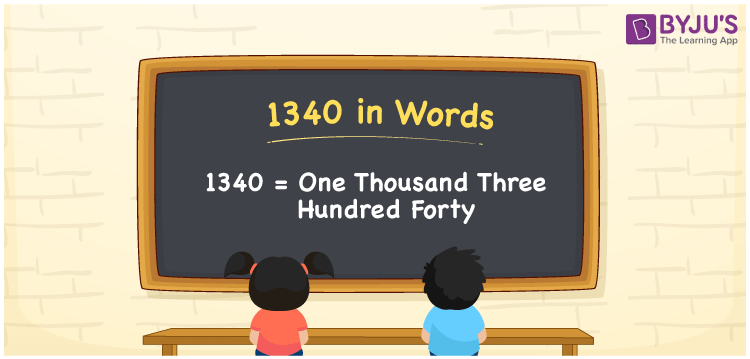# 1340 in Words

1340 in words is written as One thousand three hundred forty. In both the International System of Numerals and the Indian System of Numerals, 1340 is written as One thousand three hundred forty.

We often use 1340 as “1340 copies of this book have been sold”. 1340 represents a quantity, “how much of something” or “how many of something”. Hence, the number 1340 is a Cardinal Number.

 1340 in Words One thousand three hundred forty One thousand three hundred forty in Number 1340

## 1340 in English Words

1340 in English words is read as “One thousand three hundred forty.”## How to Write 1340 in Words?

To write 1340 in words, we shall use the place value chart. In the place value chart, put 1 in the thousands, 3 in the hundreds, 4 in the tens and 0 in the ones, respectively. Let us make a place value chart to write the number 1340 in words.

 Thousands Hundreds Tens Ones 1 3 4 0

Thus, we can write the expanded form as

1 × Thousand + 3 × Hundred + 4 × Ten + 0 × One

= 1 × 1000 + 3 × 100 + 4 × 10 + 0 × 1

= 1000 + 300 + 40 + 0

= 1340

= One thousand three hundred forty.

1340 is a natural number between 1339 and 1341.

1340 in words – One thousand three hundred forty

• Is 1340 an odd number? – No
• Is 1340 an even number? – Yes
• Is 1340 a perfect square number? – No
• Is 1340 a perfect cube number? – No
• Is 1340 a prime number? – No
• Is 1340 a composite number? – Yes

## Frequently Asked Questions on 1340 in Words

### How to write 1340 in words?

1340 in words is written as One thousand three hundred forty.

### How to write 1340 in words in the International and Indian System of Numerals?

In both, the system of numerals, 1340 in words, is written as One thousand three hundred forty.

### How to write 1340 in a place value chart?

In the place value chart, write 1 in the thousands, 3 in the hundreds, 4 in the tens and 0 in the ones, respectively.